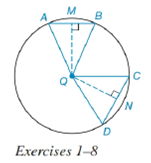Chapter 6.4, Problem 4E### Elementary Geometry for College St...

6th Edition
Daniel C. Alexander + 1 other
ISBN: 9781285195698

#### Solutions

Chapter
Section### Elementary Geometry for College St...

6th Edition
Daniel C. Alexander + 1 other
ISBN: 9781285195698
Textbook Problem
1 views

# In Exercises 1 to 8, use the figure provided.If m C D ⌢ < m A B ⌢ , write an inequality that compares m ∠ A and m ∠ C .To determine

To write:

An inequality that compares mA and mC.

Explanation

Given:

mCD<mAB and the figure given below,

Theorem:

In a circle (or in congruent circles) containing two unequal arcs, the larger arc corresponds to the larger central angle.

Calculation:

Using the theorem.

Since the arcs are unequal i.e. mCD<mAB and from the given figure.

The angle corresponding to arc mCD is mCQD and the angle corresponding to mAB is mAQB, we conclude that mCQD<mAQB.

Now considering the AQB, AQ¯=QB¯ as they are both radii.

Hence, AQB is an isosceles triangle.

Thus, the base angles are equal i.e. mQAB=mQBA.

The sum of all the angles of a triangle equals 180°. So mQAB+mAQB+mQBA=180°.

Substituting, mQAB=mQBA,

mQAB+mQAB=180°mAQB

Simplifying,

mAQB=180°2mQAB

Similarly, in QCD, CQ¯=QD¯ as they are both radii

### Still sussing out bartleby?

Check out a sample textbook solution.

See a sample solution

#### The Solution to Your Study Problems

Bartleby provides explanations to thousands of textbook problems written by our experts, many with advanced degrees!

Get Started